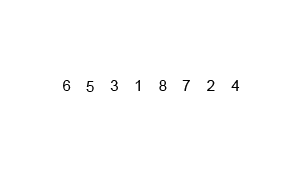# 算法入门 - 归并排序ES_her0

• 递归地把当前序列平均分割成两半（也可以不用递归）

• 保持元素顺序的同时将上一步得到的子序列集成到一起• 将列表分割成单个元素

• 创建 2 个指针，一个指向左边的部分，一个指向右边，当较小的元素被取出时，指针才会移动。一直持续到每个指针都指向队尾

#### 实现

public static void merge_sort_recursive(int[] arr, int[] result, int start, int end) {	if (start >= end)		return;	int len = end - start, mid = (len >> 1) + start;	int start1 = start, end1 = mid;	int start2 = mid + 1, end2 = end;	merge_sort_recursive(arr, result, start1, end1);	merge_sort_recursive(arr, result, start2, end2);	int k = start;	while (start1 <= end1 && start2 <= end2)		result[k++] = arr[start1] < arr[start2] ? arr[start1++] : arr[start2++];	while (start1 <= end1)		result[k++] = arr[start1++];	while (start2 <= end2)		result[k++] = arr[start2++];	for (k = start; k <= end; k++)		arr[k] = result[k];}public static void merge_sort(int[] arr) {	int len = arr.length;	int[] result = new int[len];	merge_sort_recursive(arr, result, 0, len - 1);}## 评论